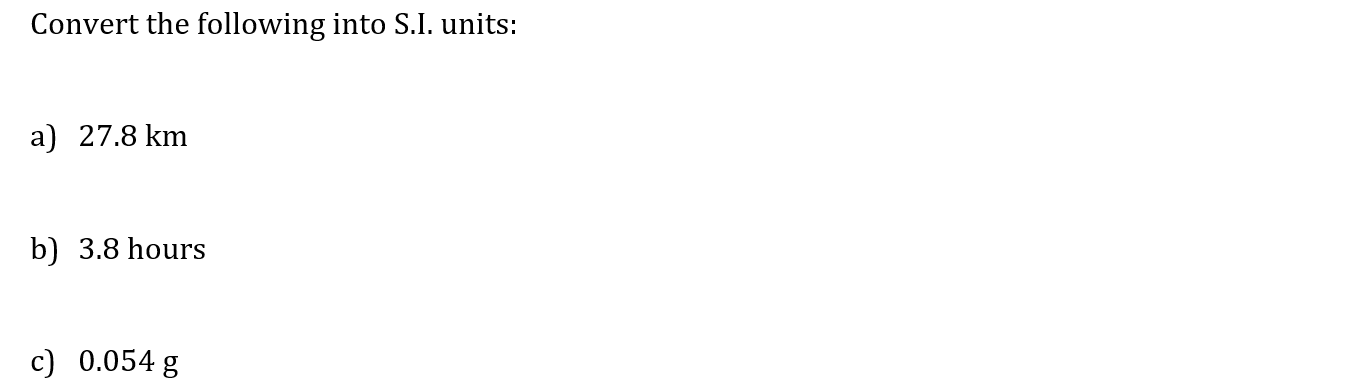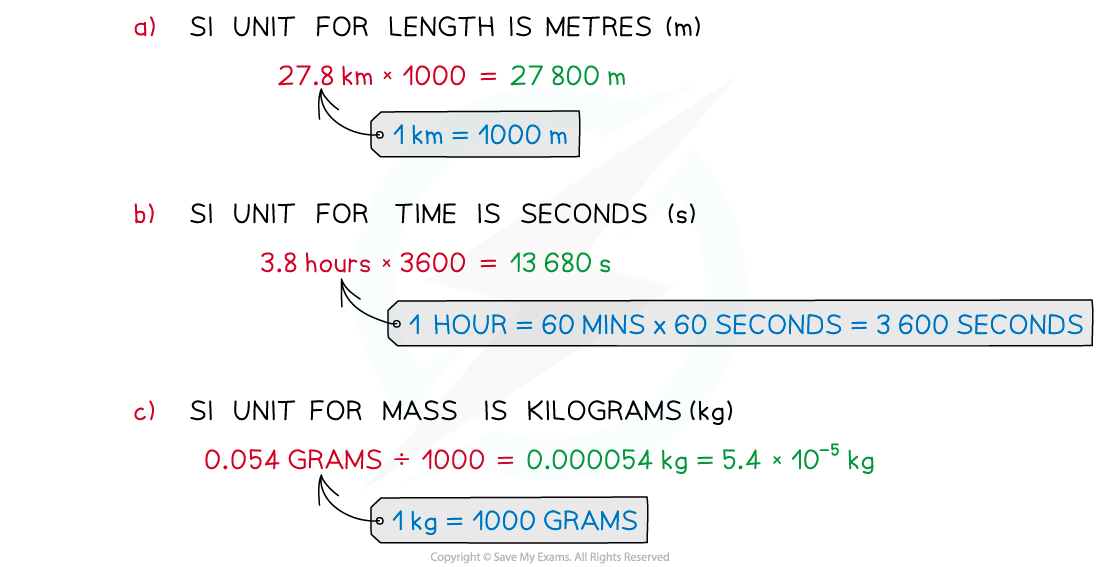# CIE A Level Maths: Mechanics复习笔记1.1.2 Fundamental Units

### Fundamental Units

What are fundamental units?

• Fundamental units or S.I. units are the international standardised units used around the world
• The three main units you will be using are:
• Length measured in metres (m) (also called displacement)
• 1 km = 1000 m, 1 m = 100 cm, 1 cm = 10 mm
• Time measured in seconds (s)
• 1 hour = 60 minutes, 1 minute = 60 seconds
• Mass measured in kilograms (kg)
• 1 kg = 1000 g, 1 g = 1000 mg
• All other quantities can be measured in units derived from S.I. units

#### Worked Example#### Exam Tip

• Make sure you fully understand the definitions of all the words in this section so that you can be clear about what your exam question is asking of you.
• Make sure you are confident converting between fundamental S.I Unitse.g.    17 minutes and 42 seconds = 1062 seconds

2.4 km = 2400 m

86000 mg = 0.086 kg.

• Watch out for exam questions using non S.I. units such as feet and inches or miles per hour.
• Remember mass (measured in kg) is not a weight (a force measured in Newtons).